Click to Chat

1800-1023-196

+91-120-4616500

CART 0

• 0

MY CART (5)

Use Coupon: CART20 and get 20% off on all online Study Material

ITEM
DETAILS
MRP
DISCOUNT
FINAL PRICE
Total Price: Rs.

There are no items in this cart.
Continue Shopping
`        I have doubt is friction give me all general formulas plzzz which r imp for lesson friction`
one year ago

```							 Types of FrictionThere are four types of friction.(a) Static Friction   (b) Kinetic Friction     (c) Rolling Friction      (d) Fluid Friction(a)Static friction:-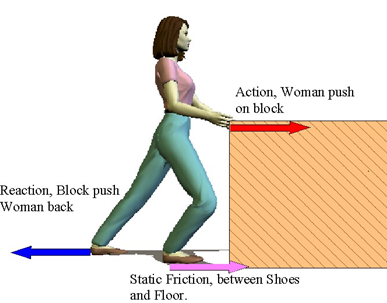Static friction is the force of friction between two surfaces so long as there is no relative motion between them. It is always equal to the applied force. The static frictional forces are incorporated in the following inequality.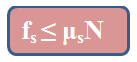The magnitude of static friction fs (static frictional force) has a maximum value fs,max  that is given by,fs,max  = µsNHere µs is the coefficient of kinetic friction and N is the normal force.So, coefficient of static friction, µs= fs,max /NStatic friction is always equal to the apllied force. It will be observe that value of static friction increases to certain maximum value, beyond which if the applied force is increased body starts moving. This maximum value of force of friction is called limiting friction.Limiting friction is the maximum value of force of friction between two surfaces so long as there is no relative motion between them.(b) Kinetic friction:- Kinetic friction is the force of friction which comes into play between two surfaces when there is some relative motion between them. The magnitude of force of kinetic friction fk (kinetic frictional force) is proportional to the normal force N.So,Here µk is the coefficient of kinetic friction.Thus, coefficient of kinetic friction, µk = fk/N  Laws of limiting friction:-(a) The direction of force of friction is always opposite to the direction of motion.(b) The force of limiting friction depends upon the nature and state of polish of the surfaces in contact and acts tangentially to the interface between the two surfaces.(c) The magnitude of limiting friction ‘F’ is directly proportional to the magnitude of the normal reaction R between the two surfaces in contact, i.e.,F∝R(d) The magnitude of the limiting friction between two surfaces is independent of the area and shape of the surfaces in contact so long as the normal reaction remains the same.(c) Rolling friction:- Force of friction which comes into play, between two surfaces, while one is rolling over the other is called rolling friction.  Rolling friction is similar to kinetic friction.So,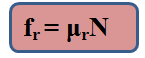Here µr is the coefficient of rolling friction and N is the normal force.(d) Fluid friction:-Fluid friction is the opposing force which comes into play when a body moves through a fluid.Cause and Direction of Rolling Friction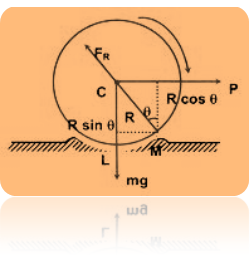A wheel of radius R rolling without sliding on a flat surface will experience a resistance due to the very small local deformation that takes place, which is sometimes elastic sometimes inelastic, i.e., a kind of ridge is formed in front of the wheel as shown exaggerated in the figure. This gives rise to the force FR, whose line of action passes through the center C of the wheel and P the horizontal force necessary to force the wheel to topple over the point M, the total clockwise torque acting on the wheel about M must be more than or marginally more than the total anticlockwise torque about M. F = Frictional forceTherefore,P × R cos θ > mg × R sin θP > mg tan θThe value of "tan θ" is called the coefficient of rolling friction (μg). This value does not depend upon R. If the two surfaces are absolutely rigid, then no ridge will be formed and q will be zero i.e., coefficient of rolling friction will be zero.Typical values are μR = 0.006 for steel and 0.02 - 0.04 for rubber tiers on concrete surfaces. Rolling friction is very small compared to the sliding friction. In case of pure rolling μR = 0.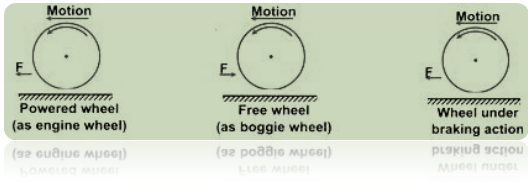The value of angle of friction and that of angle of repose are same and the tangents of both of them is equal to the co-efficient of friction.												When a body slides down an inclined plane, whose angle of inclination with the horizontal is equal to angle of repose, it moves with uniform velocity.												Sliding friction and rolling friction are independent of velocity.												Fluid friction depends upon velocity. It increases with an increase in velocity.												Force of limiting friction does not depend upon the size and shape of surface in contact.												Coefficient of kinetic friction is less than coefficient of static friction i.e.,µk ?µs																Co-Efficient of FrictionAccording to the law of limiting friction,F ∝  ROr F = μR           ............ (1)where μ is a constant of proportionality and is called the coefficient of limiting friction between the two surfaces in contact.From (1),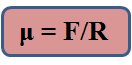Hence coefficient of limiting friction between any two surfaces in contact is defined as the ratio of the force of limiting friction and normal reaction between them. The value of μ depends on(i) nature of the surfaces in contact i.e., whether dry or wet; rough or smooth; polished or not polished.(ii) material of the surfaces in contact. For example, when two polished metal surfaces are in contact, μ ≈ 0.2, when these surfaces are lubricated, μ ≈ 0.1. Between two smooth wooden surfaces, μ varies between 0.2 and 0.5. Obviously, μ has no units. When a body is actually moving over the surface of another body, we place F by Fx, the kinetic friction, and μ and μk.Therefore,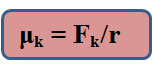μk is then called the coefficient of kinetic or dynamic friction. As Fk k is always less than μ i.e. coefficient of kinetic or dynamic friction is always less than the coefficient of limiting friction.Table gives the values of coefficient of limiting/kinetic friction between some pairs of materials:									S.No.									Surface in contact									Coefficient of limiting friction									Coefficient of kinetic friction													1.									Wood on wood									0.70									0.40													2.									Wood on leather									0.50									0.40													3.									Steel on Steel (mild)									0.74									0.57													4.									Steel on Steel (hard)									0.78									0.42													5.									Steel on Steel (greased)									0.10									0.05			 						Angle of Friction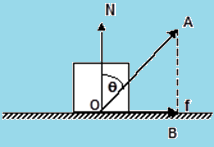The angle made by the resultant reaction force with the vertical (normal reaction) is known as the angle of the friction.  Now,  in the triangle OAB,AB/OB = cotθSo, OB = AB/ cotθ            = AB tanθOr, tanθ = OB/AB              = f / NSo, tanθ = f / N  = µsAngle of ReposeIt is the angle which an inclined plane makes with the horizontal so that a body placed over it just begins to slide of its own accord.Consider a body of mass m resting on an inclined plane of inclination θ . The forces acting on the body are shown – Ff being the force of friction. If friction is large enough, the body will not slide down.Along x: mg sin θ – f = 0          Along y: N –mg cosθ = 0                 i.e. N = mg cos θ and f = mg sin θ Thus, f ≤ µsN  gives,mg sin θ ≤ µs mg cosθ So, tan θ ≤ µs. This signifies, the coefficient of static friction between the two surfaces, in order that the body doesn’t slide down.When θ is increased, then  tan θ >  µ . Thus sliding begins, and the angle θr = tan-1µ. This angle is known as the angle of repose.
```
one year ago
Think You Can Provide A Better Answer ?

## Other Related Questions on Physical Chemistry

View all Questions »### Course Features

• 731 Video Lectures
• Revision Notes
• Previous Year Papers
• Mind Map
• Study Planner
• NCERT Solutions
• Discussion Forum
• Test paper with Video Solution### Course Features

• 141 Video Lectures
• Revision Notes
• Test paper with Video Solution
• Mind Map
• Study Planner
• NCERT Solutions
• Discussion Forum
• Previous Year Exam Questions